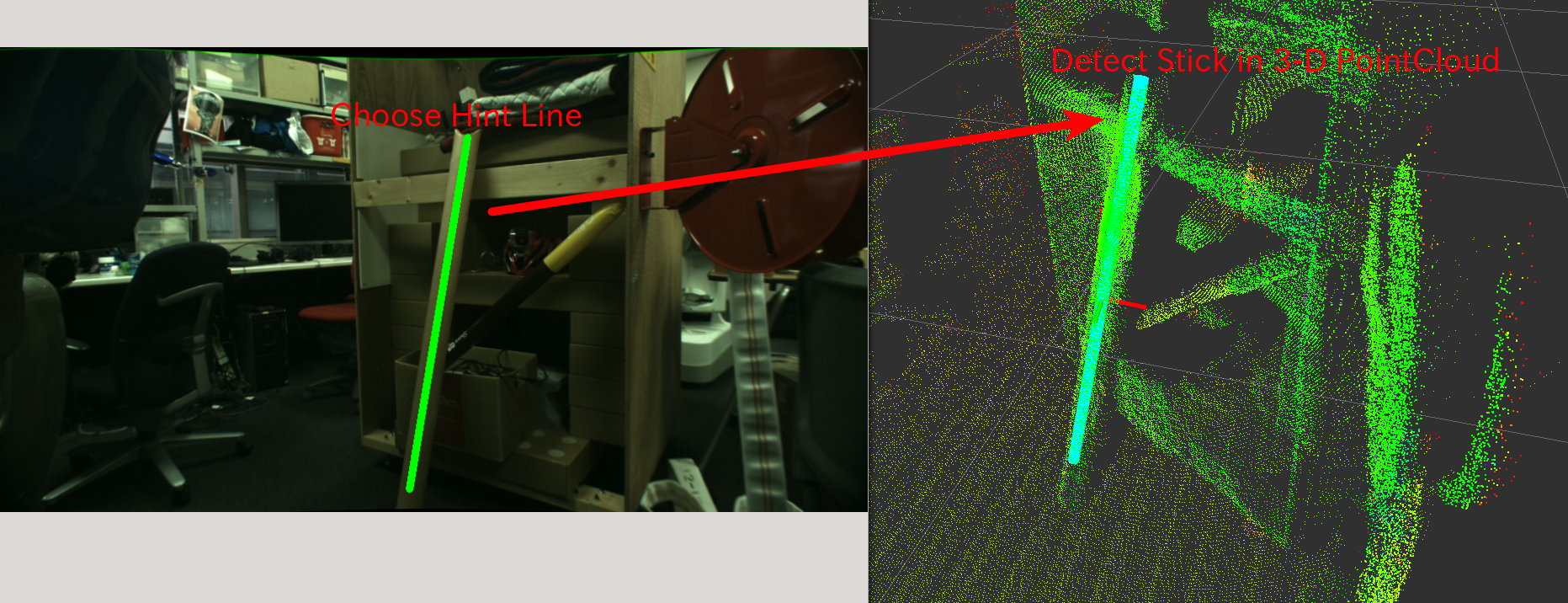# HintedStickFinder¶Detect a cylinder from pointcloud and line in 2-D image as hint.

## Subscribing Topic¶

• ~input (sensor_msgs/PointCloud2)

Input pointcloud

• ~input/camera_info (sensor_msgs/CameraInfo)

Camera parameter where hint line is defined

• ~input/hint/line (geometry_msgs/PolygonStamped)

Hint line described in 2-D image.

Only the first and second points in the polygon will be used.

## Publishing Topic¶

• ~debug/line_filtered_indices (pcl_msgs/PointIndices)

Indices of input pointcloud which is filtered by hint line.

• ~debug/line_filtered_normal (sensor_msgs/PointCloud2)

Normal pointcloud of filtered pointcloud.

This topic is advertised but not published for now.

• ~debug/cylinder_marker (visualization_msgs/Marker)

Marker topic to visualize detected stick

• ~output/cylinder_pose (geometry_msgs/PoseStamped)

Pose of detected stick.

• ~output/inliers (pcl_msgs/PointIndices)

Inliers of detected stick.

• ~output/coefficients (pcl_msgs/ModelCoefficients)

Coefficients of detected stick. The coefficients are [cx, cy, cz, dx, dy, dz, r, h].

## Parameters¶

• ~min_radius (Double, default: 0.05)

• ~max_radius (Double, default: 0.2)

Minium and maximum radius of cylinder fitting in meters.

• ~filter_distance (Double, default: 0.2)

Maximum distance in meters from hint line to points which can be cylinder candidate.

• ~outlier_threshold (Double, default: 0.01)

Outlier threshold in cylinder fitting in meters.

• ~max_iteration (Integer, default: 100)

Maximum number of iterations in cylinder fitting

• ~eps_angle (Double, default: 0.1)

This parameter is not used for now.

• ~min_probability (Integer, default: 0.8)

Required minimum probability of cylinder fitting

• ~cylinder_fitting_trial (Integer, default: 3)

The number of cylinder fitting trials when no cylinder is found

• ~min_inliers (Integer, default: 10)

Minimum number of inliers in cylinder fitting.

• ~eps_2d_angle (Double, default: 0.1)

Maximum allowable angle difference in radians between hint line and detected stick. This evaluation is done in 2-D coordinate system.

Parameters above can be changed by dynamic_reconfigure.

• ~not_synchronize (Boolean, default: False)

Do not synchronize ~input, ~input/camera_info and ~input/hint/line if this parameter is True. ~input/camera_info and ~input/hint/line are stored in nodelet and latest of the messages are used for new ~input pointcloud.

• ~use_normal (Boolean, default: False)

Do not run normal estimation inside of the nodelet and normal fields of ~input are used instead.

## Sample¶

roslaunch jsk_pcl_ros sample_hinted_stick_finder.launch